## Introduction

Low cost and high efficiency solar cells are an important alternative to fossil fuel based energy. Mono-crystalline silicon (c-Si) is undoubtedly the material of choice in photovoltaic applications due to its high natural abundance, low cost, reliability, excellent optical/electronic properties and chemical/radiation hardness1,2,3,4. However, there is continuous demand for thinner, lighter and more efficient solar cells. Present commercial screen-printed c-Si cells are typically 150–180 μm thick, enabling large absorption of the solar spectrum over the silicon bandwidth and obtain 17–20% energy conversion efficiency in volume production1,5,6. While reducing the thickness of c-Si can decrease the absorption significantly for thickness below ~50 μm, it has been shown that it leads to a higher voltage7,8,9 with the added benefit of reduced material costs10,11,12. As the cell thickness decreases, the diode saturation current decreases, resulting in the increase in voltage. In order to compensate for the low absorption of ultra-thin (<30 μm) c-Si cells, special light management schemes were developed13,14,15,16,17. Various light trapping schemes were proposed and demonstrated in recent years to enhance absorption in thin-film geometry based on top and/or bottom diffractive/plasmonic light scattering patterns18,19,20,21,22,23,24,25,26. In our previous works we have shown light trapping in ultra-thin silicon solar cells and demonstrated significant enhancement in energy conversion efficiency in a functional cell for the first time27,28. However, light trapping solar cells must also ensure maximum charge carrier collection in order to fully benefit from the increase in photon absorption. Maximizing photon absorption without efficient electron collection is not adequate to obtain higher efficiency. An electronic device physics model which includes light trapping within functional cell geometries is missing from the present literature. Thus, there is a need for a unified photon-electron harvesting scheme that enables the design of high efficiency, functional, commercial grade solar cells. This will translate to enormous material cost savings (greater W/g silicon utilization) and provide other mechanical attributes such as light-weight and flexibility to the resultant solar modules. Here, we report a unified electromagnetic and electronic device physics design approach which maximizes the combined photon-electron harvesting. The spatial absorption profile of the light trapping solar cell predicted from finite difference time domain (FDTD) simulations define the charge carrier generation in the following electronic device simulation. The predicted short circuit current (Jsc), open circuit voltage (Voc) and efficiency (η) from an optimized doping profile and carrier life time closely match experimental observations in bare as well as light trapping cell geometries.

## Results and Discussion

### Light trapping c-Si solar cell optical responses

Figure 1(a) shows the chosen light trapping cell architecture. The light trapping scheme is composed of top nanostructured diffractive optical element coated with anti-reflection coating coupled to an optical cavity which enhances absorption via a composite phenomenon: reduction in reflection, path length enhancement via forward diffraction and trapping light into the silicon waveguide and cavity modes. An integrated 78% absorption with respect to the AM1.5G spectrum is numerically predicted inside the 3 μm thick light trapping cell as shown in Fig. 1(b). This constitutes 117% absorption enhancement compared to a bare wafer of same thickness which absorbs only 36% of the integrated solar spectrum. For the top diffractive pattern a 2D hexagonal Bravais lattice was chosen based on the design reported in our earlier publications27,29. Figure 1(c) presents the band diagram of the corresponding n-p-p + junction under illumination when is connected to an external load. The lack of the acceptor impurity concentration at the base contact causes the minority carriers to recombine more easily, which is considered as one of the main sources of the voltage loss in a solar cell19. Hence, a heavily p-doped region at the base electrode improves the open circuit voltage. The band tilts downwards in the n region and upwards in the p region due to the positive and negative charge movement, respectively. Under illumination, the number of generated excess electrons is equivalent to the number of generated excess holes; hence the Fermi energy difference at the n and p sides, εfn−εfp becomes closer to the band gap energy by increasing the numbers of excess minority carriers which leads to higher current density. This phenomenon is further described in the following section.

Figure 2(a) shows the 3D FDTD predicted light trapping pattern optimization for a constant silicon thickness (3 μm). The wavelength integrated absorption is maximized as a function of 2D hexagonal lattice period (P), diameter to period ratio (D/P) and relief depth (RD) for a constant ARC layer (SiO2/SiN = 50/35 nm). According to Fig. 2(a), the optimized light trapping pattern which enables the maximum absorption of 78% integrated solar spectrum (400–1100 nm) (marked as star on Fig. 2(a)) defines the posts dimension of period 500 nm, diameter/period 0.6 and relief depth 140 nm. Further based on this optimized pattern, the silicon absorption as a function of silicon thickness is studied and shown in Fig. 2(b). It can be seen that for this light trapping design silicon thickness of >15 μm is needed in order to absorb >90% integrated AM1.5 solar spectrum. Figure 3(a) compares the FDTD predicted absolute absorption of a 3 μm thick bare and a light trapping cell as a function of wavelength. Significant absorption enhancement can be observed in the light trapping cell especially near the band edge where bare silicon absorption is weak. Furthermore, narrow Fabry-Perot cavity resonances are observed in the light trapping cell which correspond to the presence of the 0th and higher order cavity modes of the thin cell. The FDTD predicted 2D absorption profiles (Pabs) of bare and light trapping cells in a strong (λ = 461 nm) and weak (λ = 977 nm) absorption regimes are shown in Fig. 3(b). Absorbed power is obtained by applying equation (1), which is derived from the divergence of the Poynting vector formula as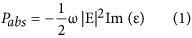According to the equation (1), the energy absorption of a monochromatic light is directly proportional to the electric field intensity which mandates field enhancement for higher absorption. As predicted, the band edge photons (λ = 977 nm) are more strongly absorbed in the light trapping cell compared to the bare cell due to tight field confinement and path length enhancement via diffraction/scattering. There is significant enhancement in absorbed power between light trapping and bare cells which can be noticed by comparing Fig. 3(b), top and bottom. This fact is further supported by the wavelength integrated generation rate (g) in Fig. 3(c). The number of electron-hole pairs generated by absorbed photons as a function of position integrated over a specific wavelength range in the device is defined as the wavelength integrated charge carrier generation rate (g). From Fig. 3(c), significantly enhanced charge carrier generation can be observed for the light trapping cell due to the stronger photon absorption substantially deep inside the wafer. Subsequently, the FDTD predicted 3D generation rate data is imported into the numerical electronic modeling (Lumerical DEVICE, Lumerical Inc.) that makes the bridge between electromagnetic absorption and electronic device performance predictions. Since the actual cell is longer than one unit cell due to the lateral p-n junction (Fig. 1(a)), the FDTD predicted one unit cell generation rate, which is calculated with the periodic boundary condition along the x-y directions, is copied along the length of the device to cover the distance between two contacts.

### Electronic device modeling

The quasi fermi energy levels, electron-hole densities and recombination losses of the cell were extracted from the electronic simulations in order to understand the fundamental gain and loss mechanisms. For a fair comparison, both bare and light trapping cells are assumed to be perfectly passivated with reasonably low surface recombination velocity (SRV) SRV = 10 m/cm. A low SRV isolates the effects of recombination and electron-hole generation processes. Figure 4(a) shows the chosen doping profile of the 3 μm silicon cell with and without light trapping. The p and n regions are shown as gradient of the doping concentration using two opposite color schemes. Figure 4(b) compares the electron density of a 3 μm bare and light trapping cells. The light trapping cell shows a higher absolute electron (hole) density due to the higher absolute quasi fermi energy levels which, in turn, originates from the higher absorption. The electron density in non-equilibrium conditions (i.e. under illumination as a function of the quasi fermi energy) is given by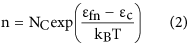where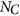is the effective density of state in the conduction band, εfn and εc are electron quasi fermi energy and conduction band energy respectively. The carrier density is highly sensitive to any small variation in quasi fermi energy level. As can be seen in Fig. 4(b), the electron density within the n-type region of the light trapping cell is significantly higher than that of the bare cell. The n and p quasi fermi energy difference (εfn − εfp) is a measure of the deviation from equilibrium. Under non-equilibrium conditions, i.e. under illumination, the current density can be written as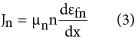where μn is the electron mobility. The electron density in the non-equilibrium condition is n = n0 + Δn where n0 is the donor impurity concentration (at equilibrium) and Δn is the excess minority carrier concentration that is generated under illumination. The higher value of charge density, in conjunction with higher quasi fermi energy, leads to a greater electron current density. Figure 4(c) illustrates the electron current density enhancement in light trapping cell with respect to the bare cell. Current density that is proportional to the gradient of the quasi fermi level, shows the flow of photo generated carriers towards ohmic contacts and through the external circuit. The concentration of holes and corresponding hole current density can be explained in a similar fashion. For fair comparison, both electron density and current density (Fig. 4(b,c)) are integrated over the corresponding predicted I-V curve shown in the following.

Apart from the light absorption enhancement in the light trapping cell, which leads to higher internal current density as demonstrated above, it is also important to investigate the mechanisms of loss in order to improve charge collection and overall cell efficiency. The surface recombination velocity (SRV), which is one of the main sources of voltage loss and low short circuit current, defines the carrier recombination rate at the silicon interfaces due to the silicon dangling bonds. Figure 5(a) shows the predicted variation in Jsc as a function of SRV for bare and light trapping cells. Although, a lower SRV (1–100 cm/s)30,31,32,33 produces higher Jsc, achieving such low SRV on a patterned surfaces of a light trapping cell requires excellent surface passivation. The impact of bulk and surface recombination on the cell performance can be described by the effective lifetime, given by30,34,35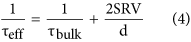where d is the cell thickness. According to the equation (4), in lower cell thicknesses, SRV becomes more significant than the bulk lifetime. Thus a good multi-functional passivation layer on the patterned top surface which also functions as an anti-reflection coating makes a significant difference in device performance due to both photon absorption enhancement and carrier lifetime improvement. The SRV further influences the open circuit voltage which is defined in equation (5) for a solar cell made on a p-type wafer as36,37,38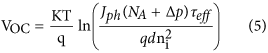where Jph is photocurrent density, NA is the acceptor concentration, ∆n and ∆p are the electron and hole excess minority carrier concentration respectively which are equivalent under the illumination in solar cells and ni is the intrinsic carrier concentration. Since SRV is inversely related to the lifetime, τeff (Equation (4)), it reduces the open circuit voltage as can be observed in Fig. 5(b) and ultimately the energy conversion efficiency of the cell. Both the current density and the voltage (Fig. 5(a,b) of the light trapping cell decreases significantly compared to that of the bare cell as SRV increases. This sharp fall can be explained by higher surface recombination due to the surface texturing in light trapping cells. Figure 5(c,d) shows the short circuit current density vs doping concentrations for boron and phosphorus regions in bare and light trapping cells respectively for the surface recombination velocity of 1000 cm/s. A SRV of 1000 cm/s is chosen for the doping concentration studies since lower SRVs can only be obtained by thermal silicon oxide growth which is not applicable in this case due to polymeric substrates of fabricated microbar cells29,39. A significant enhancement in Jsc can be observed in the light trapping cell compared to the bare cell. The phosphorus and boron doping concentration studied regime is 6e + 20–1e + 21 cm−3 and 3.7e + 20–4.3e + 20 cm3, respectively. These variation ranges are based upon the pre-deposition furnace temperature of 900 °C–1050 °C in which the cells are exposed to the infinite constant sources40. Since our studied cell is ultrathin, 3 μm, compared to conventional silicon solar cell thickness (150–180 μm) the drive-in diffusion process that is occurred at higher temperature (T > 1000 °C) in the absence of the dopant source in order to increase the junction depth is not considered in this study. These results imply that lower doping concentration leads to higher current densities, at the studied doping concentration regime41 which also can be shown theoretically by: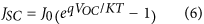where J0 is the dark current which flows through the solar cell when a bias is applied in the dark and in low level injection is given by: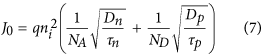Dn, Dp are the electron and hole diffusion coefficients, τn, τp are the lifetime of electron and hole, respectively. Comparing Eq. (6) and Eq. (7) we can conclude that a lower doping concentration (NA, ND) leads to higher J0 and consequently higher Jsc as observed in the numerical simulation in Fig. 5(c,d).

Deep level or Shockley-Read-Hall (SRH) and Auger recombination are the other two carrier loss mechanisms which severely affect solar cell performance. The more heavily doped material leads to higher auger recombination and the material with more defect states has higher Shockley-Read-Hall recombination. These recombination mechanisms are defined as42: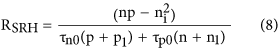where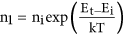,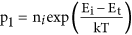, τn0, τp0 are SRH electron and hole lifetime,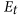is trapping energy level, and: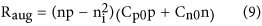where Cp0, Cn0 are auger coefficients which have inverse quadratic dependence upon carrier concentration (unit cm6/s). From Fig. 6(a,b) it can be seen that the light trapping cell has a higher Auger and Shockley-Read-Hall recombination rate within the posts due to the generation of higher excessive minority carriers and higher defect density, respectively. Both recombination rates are integrated over the respective I-V curve (Fig. 7(b)) of bare and light trapping cells in order to demonstrate the overall response. The light trapping nano-patterning introduces more localized doping distribution and more defects to the silicon cell which leads to higher auger and SRH recombination. However, enhanced absorption and carrier generation in light trapping cells compensates these recombination losses and gives a higher overall cell efficiency. Further, this work shows that the device performance can be enhanced by minimizing recombination losses via optimum doping profile and surface passivation.